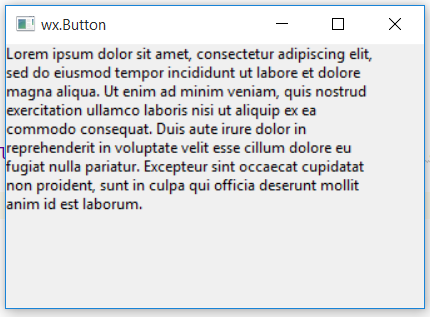Open In App

# wxPython – Wrap() function in wx.StaticText()

In this article we are going to learn about Wrap() function associated with wx.StaticText class of wxPython. Wrap() functions wraps the controls label so that each of its lines becomes at most width pixels wide if possible (the lines are broken at words boundaries so it might not be the case if words are too long).

If width is negative, no wrapping is done. Note that this width is not necessarily the total width of the control, since a few pixels for the border (depending on the controls border style) may be added.

Syntax: wx.StaticText.Wrap(self, width)

Parameters:

ParameterInput TypeDescription
widthintwidth for wrapping.

Code Example:

 `import` `wx`` ` ` ` `class` `Example(wx.Frame):`` ` `    ``def` `__init__(``self``, ``*``args, ``*``*``kwargs):``        ``super``(Example, ``self``).__init__(``*``args, ``*``*``kwargs)``        ``self``.InitUI()`` ` `    ``def` `InitUI(``self``):``        ``self``.locale ``=` `wx.Locale(wx.LANGUAGE_ENGLISH)``        ``self``.pnl ``=` `wx.Panel(``self``)`` ` `        ``bmp ``=` `wx.Bitmap(``'right.png'``)``        ``# CREATE STATICTEXT AT POINT (20, 20)``        ``self``.st ``=` `wx.StaticText(``self``.pnl, ``id` `=` `1``, label ``=``"Lorem ipsum ... laborum."``, pos ``=``(``0``, ``0``),``                                 ``size ``=` `wx.DefaultSize, style ``=` `0``, name ``=``"statictext"``)`` ` `        ``# WRAP TEXT IN A PARTICULAR WIDTH``        ``self``.st.Wrap(``300``)`` ` `        ``self``.SetSize((``350``, ``250``))``        ``self``.SetTitle(``'wx.Button'``)``        ``self``.Centre()`` ` `def` `main():``    ``app ``=` `wx.App()``    ``ex ``=` `Example(``None``)``    ``ex.Show()``    ``app.MainLoop()`` ` ` ` `if` `__name__ ``=``=` `'__main__'``:``    ``main()`

Output Window: